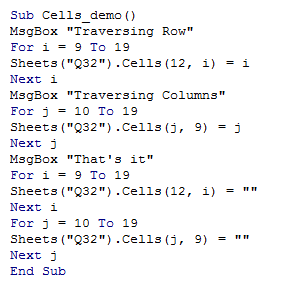## VBA cells object in Excel

In the previous chapters, Range object was discussed.It is simply used to access a contigous range of cells.

The Cells object is used to access a single cell.

The Range object access a cell using its name, like A1,G7 and so on, whereas, Cells object access a cell with the help of Row and Column Index.

The Cells() comes to help, when a row has to be traversed along, with a For loop.

The Range() will be useful to traverse down a column, whereas cells() will be useful for both rows and columns.

The Syntax:

Sheets("sheet_name").Cells(row_index,col_index)

The following example illustrates this:

1. Sub Cells_demo()
2. MsgBox "Traversing Row"
3. For i = 9 To 19
4. Sheets("Q32").Cells(12, i) = i
5. Next i
6. MsgBox "Traversing Columns"
7. For j = 10 To 19
8. Sheets("Q32").Cells(j, 9) = j
9. Next j
10. MsgBox "That's it"
11. For i = 9 To 19
12. Sheets("Q32").Cells(12, i) = ""
13. Next i
14. For j = 10 To 19
15. Sheets("Q32").Cells(j, 9) = ""
16. Next j
17. End Sub

As we saw from the demo, The cells method can be used to read or write data along a single row, (L9,M9,N9 and so on).

Whereas with Range, it is not easy to do the same functionality, though, it is more advantageous to read or write along a single column.

The VBA screenshot is as shown below: